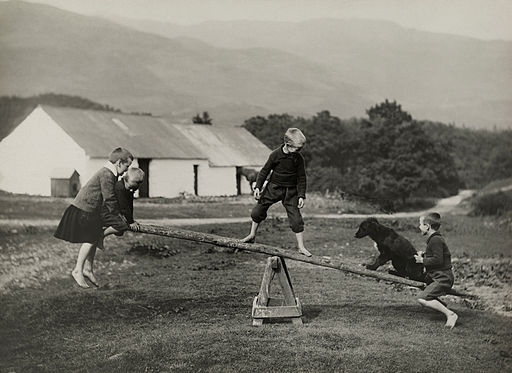# Difference Between Torque and Torsion

## Main Difference – Torque vs. Torsion

Torque and torsion are both related to turning effects experienced by a body. The main difference between torque and torsion is that torque describes something that is capable of producing an angular acceleration, whereas torsion describes the twist formed in a body due to a torque.

## What is Torque

Torque describes a force that is capable of changing an object’s angular velocity or causing torsion about an axis. The torque produced by a force is found by multiplying the perpendicular distance between the line of force and the axis of rotation. Alternatively, one could take the force component perpendicular to the shortest line between the axis of rotation and the point of application of the force, and then multiply this component by the length of the shortest line. This is depicted in the diagram below:Definition of Torque

Torque is given by$\tau$, and the shortest distance between the axis of rotation and the point of application of the force is$r$. The force is$F$ and the force component perpendicular to the shortest line between the axis of rotation and the point of application is$F_{\perp}$. Then, the torque is given by$\tau =rF_{\perp}$.

The formal definition for torque uses the cross product, and in this way torque is defined as:$\vec{\tau}=\vec{r}\times\vec{F}$

This means that the magnitude of torque can be given as$\tau=rF\mathrm{sin}\theta$, which is equivalent to the first definition for torque that was given.

In everyday situations, torques are what we use to create turning effects or to produce twisting. When you use a spanner to tighten a nut, you are applying a torque. When you turn a steering wheel, you apply two antiparallel forces on the wheel that create torques. In a see-saw, torques are produced at each end by the weights of people on it.A see-saw works because of torques

## What is Torsion

Torsion describes a “twisting” effect, where different parts of an object undergo different angular displacements (referred to as the “angle of twist”) about the rotational axis. To create torsion, a torque is necessary.Torsion describes a twisting effect.

Torsion happens when the torque causes a shear stress to be applied on the body. When the shear stress applied to different parts of the body is different,  they undergo different amounts of angular displacements. The maximum angle of twist occurs at the ends of the object while the middle experiences a zero angle of twist. It can be shown that for an object with a uniform cross-section under torsion by a torque$T$, the maximum angle of twist$\theta$ is given by:$\theta=\frac{TL}{JG}$

Here,$L$ is the length of the object,$J$ is the quantity known as “torsion constant“, and$G$ is the shear modulus of the material.

## Difference Between Torque and Torsion

### What it Describes

Torque is something that has the ability to create an angular acceleration.

Torsion is a twist produced due to torsion.

Image Courtesy

“A force is applied to a particle free to rotate about a fixed axis. Force is shown decomposed into perpendicular and parallel components…” by StradivariusTV (Own work) [CC BY-SA 3.0], via Wikimedia Commons

“A dog plays on a seesaw with children in Scotland, March 1919. Photograph by William Reid, National Geographic” by Photographes du National Geographic [Public Domain], via Wikimedia Commons

“Torsion of a solid square section.” by Orion 8 (Own work) [CC BY-SA 3.0], via Wikimedia Commons